# Survival Analysis: Comparison of Survival Curves Using Historical Controls

Hypothesis: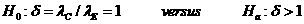Data Input: (Help) (Example)

 Input Results α δ MS r P nC N yC τ T

Note:

 Variables Descriptions α Significance level λC Hazard rate of control group λE Hazard rate of experimental group δ Minimum hazards ratio,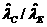, the ratio of two estimated hazard rates MS Control median survival (month) r Accrual rate (per month) nC Number of deaths observed in the Control group yC Number of patients still at risk in the Control group τ Length of the planned continuation period (month) T Length of the actual time for the new study (month) P Power of the test N Number of Patients in the Experimental group

Help Aids

Application:  The determination of the number of patients needed in a prospective comparison of survival curves, when the control group patients have already been followed for some period, following hypotheses are usually used:Procedure:

1. Enter

a)   value of α, the probability of type I error

b)   value of δ, the minimum hazards ratio, it is specified in alternate hypothesis (i.e.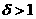)

c)   value of MS, the control median survival (month), it is estimated from existing control data

d)   value of r, the accrual rate (per month), it is estimated for future accrual

e)   value of nC, the number of deaths observed in the control, it is obtained from existing control data

f)   value of yC, the number of patients still at risk in the control, it is obtained from existing control data

g)   value of τ, the length of the planned continuation period (month)

h)   value of T, the length of the actual period for the new study (month).

1. Click the button “Calculate” to obtain the power of the test and the sample size of patients in the experimental arm.
2. Adjust the variable T (accrual time) until desire power is obtained (e.g. 80%).

Assumption:

1.      Time to survival is exponential distributed with hazard rate λ.

2.      Prospective studies are used.

3.      There is no withdrawal or losses to follow-up.

Formula:

Null hypothesis for two exponential distributions has a rejection region.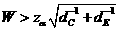(1),

where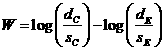,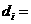number of failures observed in group i,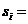total patient years of observation time in group i,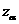is the upper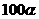percentile of the standard normal distribution.

For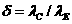, where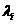is the true failure rate in group i, (1) is the critical region of size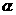for the one-sided alternative.

The power of the test for a specific value of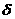is approximately(2),

where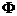is the cumulative standard normal distribution function,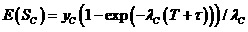(3),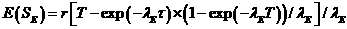(4),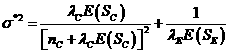(5),

nC is the number of failures observed in the control group,

Si is the total additional observation time to be observed in group i,

yC is the number still at risk in the control group,

r is the accrual rate,

T is the length of the accrual period for the new study,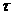is the length of the planned continuation period.

Note:  (If withdrawals are expected to occur at rate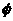, then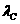and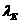in equation (3) and (4) should be replaced by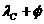and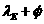, respectively.) To calculate the power of a particular study, one replacesby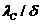and placesby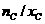, where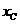is the total observation time already observed in the control group. If additional follow-up of remaining controls were not possible, one would set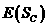to zero in (2).

Notations:

α:         The probability of type I error (significance level) is the probability of rejecting the true null hypothesis.

β:         The probability of type II error (1 – power of the test) is the probability of not rejecting the false null hypothesis.

Example:

Suppose one wishes to design a trial to compare new treatment E with control treatment C, on the basis of survival duration. Historical data for C from a recently compared trial are available: a total 100 patients, of whom 50 have already died (nC=50, yC=100-50=50), with a median survival of 12 months (MS=12). The aim is to have 80% power (P=80%) to detect a ratio of hazard rates of 1.5 (δ=1.5), using a one-sided, 5% significance level test (α =0.05) after a one-year continuation period (τ=12). Recent experience indicates that new eligible patients will arrive at the rate of three per month (r=3). The required accrual target is approximate 88 patients (N=88) with actual time about 29.3 months for the new study (T=29.3).

Reference: Dixon & Simon (1988) J Clin Epidemiol 41:1209-1213